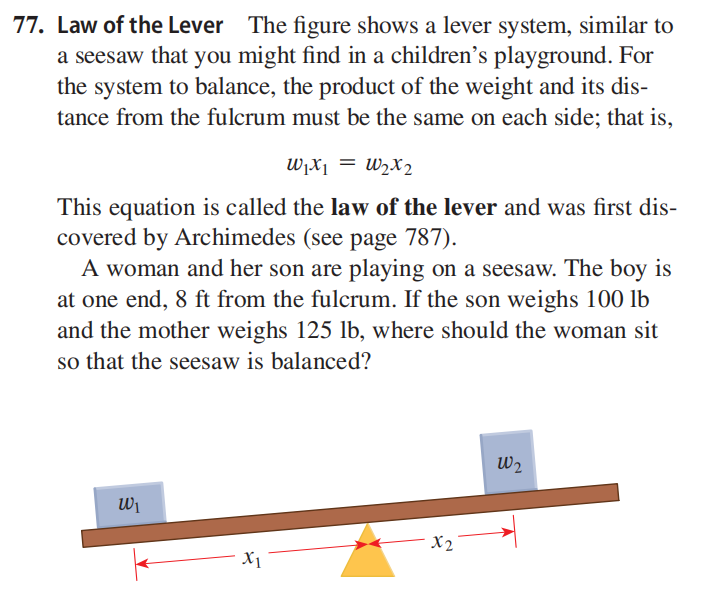### ¿Todavía tienes preguntas de matemáticas?

Pregunte a nuestros tutores expertos
Algebra
Pregunta77. Law of the Lever

The figure shows a lever system, similar to a seesaw that you might find in a children's playground. For the system to balance, the product of the weight and its dis- tance from the fulcrum must be the same on each side; that is,

$$w _ { 1 } x _ { 1 } = w _ { 2 } x _ { 2 }$$

This equation is called the law of the lever and was first dis- covered by Archimedes (see page $$787$$ ).

A woman and her son are playing on a seesaw. The boy is at one end, $$8 ft$$ from the fulcrum. If the son weighs $$100 lb$$ and the mother weighs $$125 lb$$ , where should the woman sit so that the seesaw is balanced?

$$\text { 77. } 6.4 ft \text { from the fulcrum }$$